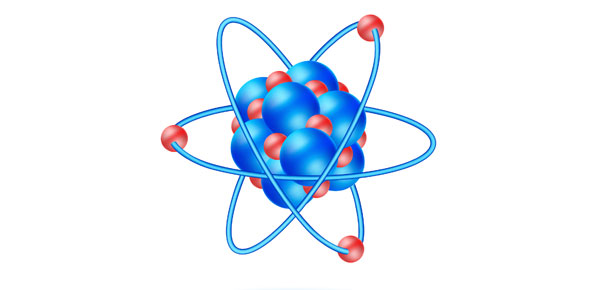# Physical Science; Ch. 11 Quiz 1

10 Questions | Attempts: 259
ShareSettingsQuiz 1 on Ch. 11; Forces in Fluids

• 1.
If you divide the force exerted on a surface by the total area of the surface, you will know...
• A.

Density

• B.

Pressure

• C.

Lift

• D.

Buoyant Force

• 2.
If you divide the mass of an object by its volume, you also know the object's
• A.

Mass

• B.

Weight

• C.

Density

• D.

Pressure

• 3.
The weight of an object that floats has the same value as the
• A.

Object's density

• B.

Objects mass

• C.

Object's volume

• D.

Buoyant force

• 4.
The concept that an increase in pressure on a confined fluid is transmitted equally to all parts of the fluid is known as
• A.

Pascal's principle

• B.

Bernoulli's principle

• C.

Archimede's principle

• D.

Mr. Thompson's principle

• 5.
The concept that the pressure in a fluid decreases as the speed of the fluid increases is known as
• A.

Pascal's principle

• B.

Kidwell's principle

• C.

Archimedes principle

• D.

Bernoulli's principle

• 6.
If you stand on one foot you ________ the pressure you exert on the ground.
• 7.
Whether an object will float or sink in a liquid depends on the _________ of the object relative to that of the liquid.
• 8.
A submerged object is easier to lift due to the ____________ force acting on the object in the upward direction opposing the downward force of gravity.
• 9.
A ship is able to float on the ocean due to ______________ principle which states that the buoyant force acting on a submerged object is equal to the weight of the volume of water displaced by the object
• 10.
An airplane is able to fly due in part to ________________ principle which states that the faster a fluid moves, the less pressure the fluid exerts.

## Related TopicsBack to top
×

Wait!
Here's an interesting quiz for you.